ISSN ONLINE(2278-8875) PRINT (2320-3765)

All submissions of the EM system will be redirected to Online Manuscript Submission System. Authors are requested to submit articles directly to Online Manuscript Submission System of respective journal.

# On The Role of Supercapacitors towards Characterization of PV Generators

 T. Belel1, S. Basu Pal2, K. Das (Bhattacharya)3, D. Mukherjee4 Department of Electronics Engineering, IIEST, Shibpur, West Bengal, India Department of Electrical Engineering, IIEST, Shibpur, West Bengal, India Department of Electrical Engineering, IIEST, Shibpur, West Bengal, India Department of Electronics Engineering, IIEST, Shibpur, West Bengal, India Related article at Pubmed, Scholar Google

Visit for more related articles at International Journal of Advanced Research in Electrical, Electronics and Instrumentation Engineering

## Abstract

A very precise and inexpensive method of I-V characterisation is possible by capacitor charging for a PV generator. Application of transient analysis on the circuit permits determination of characterization times for various capacitor sizes. Following the above trend, authors of this paper uses various sizes of supercapacitor instead of capacitor as load to PV cell and modules. This ensures smother characterization without noise and also accurate control over the characterization time. Based on a piecewise linear break-up of PV characteristic, authors have derived a much more accurate method of characterization time involving the four basic electrical parameters defining a PV curve. Experimental validation indicates excellent agreement with actual curve plotting time.

### Keywords

I-V characterisation, PV Generator, Data Acquisition System, Supercapacitors.

### I.INTRODUCTION

Several Methods to plot the I-V characteristics of a PV cell/module automatically have been published [1-5]. Such plots alone can be analysed to estimate the essential electrical PV parameters of interest .Since the deployment of large size PV arrays have currently invaded the urban scenario, the role of on-line, simple and inexpensive I-V curve tracers is expanding outside the academic laboratories also. Simple analog approaches  face several obvious constraints such as (a) Need of extrapolation in the plotting, leading to inaccurate estimate of shunt(Rsh) and series(Rs) resistances (b) Possibility of a thermal influence on the curve in case of longer periods of characterization. Hardware independent tool kits  perform forward bias I-V characterization using Lab-VIEW software depending on prior acquired data and are hence useless for real time applications. One high-end set-up designed and commissioned by the solar Laboratory of the Wroclaw University of Technology, Poland uses class A solar simulator and meets all requirements of IEC- 60904-3 and 60904-9 standards. But such a set-up is too sophisticated to suit the needs of an on-line characterisation in PV labs and workshops. PV performance models like solar Advisor Model Simulation Software used for commercial purposes are too sumptuous to be used in academic laboratories and testing levels. A recent effort by S.K. Ghosh et.al  has employed an Arduino Integrated development board (IDE) through serial bus and PLX-DAQ software for automatic and fast plotting. But the yielded characteristics are too noisy in nature to be used for parametric evaluation. Of late, there is an effort by Marwan.M.Mahmoud et al  enabling the selection of correct value of a capacitor as the load to a PV cell/module. In view of the above backdrop, the authors, in this proposition, uses a small supercapacitor bank with an Agilent Data Acquisition System (34970A) to control the characterization time and noisy co- ordinates. Next section is devoted to a step by step survey of V-I characterization using various types of load components. This is essential for an appreciation of the present methodology practiced by the authors.

### II. RELATED WORKS FOR V-I CHARACTERIZATION

II.I. Using a variable resistor:
The V-I characteristics of a solar cell/module/array can be traced simply and inexpensively by using a variable resistive load (RL) as shown in figure1.Here RL is varied from 0 to infinity to trace the curve starting from short circuit point to open circuit point respectively. However the method suffers from low accuracy as already mentioned. Also to avoid frequent human intervention required in this method, Marwan M.Mahmoud proposed a transient way of V-I characterization using capacitor .
II.II Using Capacitor as the load:
In this method the variable resistor has been replaced by a capacitive load. The capacitor voltage is automatically swept from zero to open circuit voltage by drawing current from the PV generator in a very short amount of time. In the process, respective voltage and current values are recorded by a data recorder or a computerized data acquisition system (CDAS). The required I–V curve obtained by this method is much more accurate and also it overcomes the drawbacks of the first method. However for the curve tracing of high power PV generators, it requires highly sensitive DAS with a very high scanning speed, which is a costlier proposition.
II.III Using Supercapacitor:
In this technique, tracing the I-V curve of a photovoltaic cell/module has been done using the Supercapacitors for the first time. Although Supercapacitor calls for a moderate scanning speed, a reduced device complexity than the capacitor method in conjunction with a compatible Data Acquisition System (DAS), a reasonably low curve tracing time have inspired the authors to replicate their proposition with large number & variety of PV cells and modules. The supremacy of this method over resistive method can be observed in figure 2 and figure 3. It can be seen that data points are discontinuous in resistive method, leading to incorrect determination of maximum power point.
Tracing V-I curve of a Photo-Voltaic (PV) cell, module or array from the transient analysis of charging a capacitor has been already established . The Supercapacitor method for tracing V-I curve also works on the same principle. Consider the circuit arrangement in figure 4.
A PV cell/ module give a fixed current at a fixed voltage for a particular light intensity and stable environmental conditions. With increasing voltage from zero to open circuit voltage(Voc)derived current is almost constant initially and equal to short circuit current (Isc)Then it starts to decrease and comes to zero at V=Voc A discharged Supercapacitor connected across a PV cell, module or array terminal applies zero voltage at the terminal and due to its negligible Equivalent Series Resistance (ESR) draws a current equal to Isc at the beginning of charging by the PV generator. With drawing current and accumulating charge the terminal voltage of Supercapacitor increases and following the I-V characteristic of the PV generator, the current drawn is being decreased.

### IV. ANALYSIS OF THE CURVE TRACING TIME USING SUPERCAPACITOR METHOD

Previously Marwan M.Mahmoud have given a simple expression of curve tracing time, involving Isc and Voc terms only .Although a curve nature can vary in different ways between short circuit point and open circuit point and so does the curve tracing time, the authors have approximated the V-I curve with piecewise linear approach for deriving a more appropriate expression for the curve plotting time.
The above Figure 5 represents basic V-I characteristic of a solar cell/module. Here A(0,Isc) denotes short circuit point, B(Vm,Im) denotes maximum power point, C(Voc,0) denotes open circuit point.
Short circuit point A(0, Isc ) to peak power point(Vm, Im) is approximated by the straight line-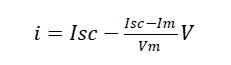(1)
Peak power point B(Vm, Im) to open circuit point C(Voc,0) is approximated by the straight line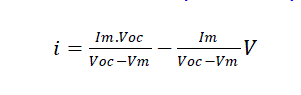(2)
For the supercapacitor,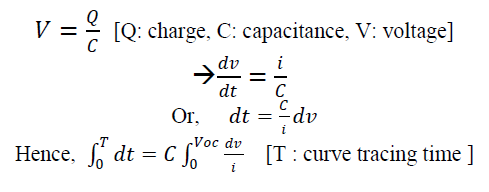Therefore,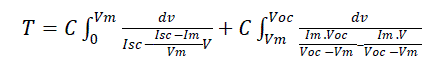(3) [Using equation 1 and equation 2]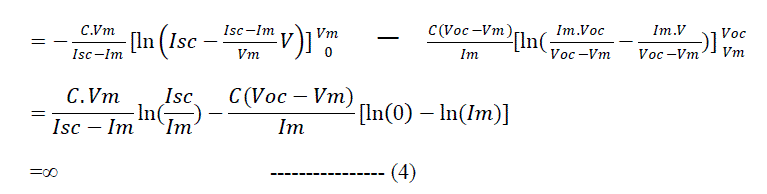This equation signifies that Supercapacitor voltage cannot reach the exact Voc point. It can be intuitively verified from figure 6 and figure 7, the charging characteristic of supercapacitor directly fed by a solar cell or module. Here we can see that both voltage change and current change with respect to time have been slowing down at the end .So it will take infinite time to reach their optimum.
But in practice it can reach more than 99% of the open circuit voltage which is fair enough for precision curve tracing. Say, Supercapacitor voltage reaches K% of the open circuit voltage. Hence, changing the limits in equation 3, we get-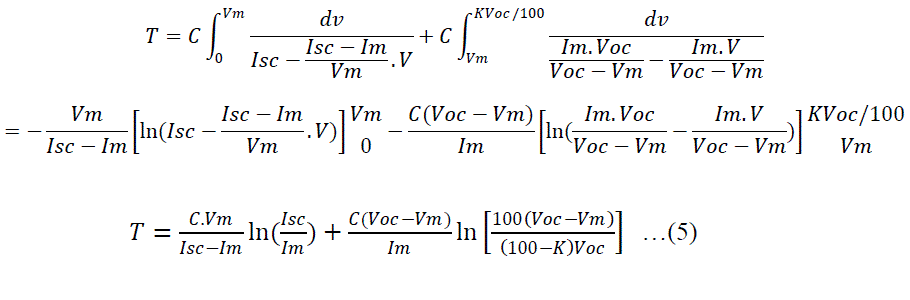### V. EXPERIMENTAL SETUP

The experimental arrangement consists of PV cell or module, Supercapacitor bank, DAS, computer, variable intensity light source, current sensing resistor (Rcs) and two switches (figure 2). The DAS connected to the computer is configured and controlled by a Graphical User Interface (GUI). DAS collects current information in terms of scaled voltage across Rcs Switch S1 is opened and S2 is closed before beginning the experiment to discharge the Supercapacitor Bank. Then S2 is opened and S1is closed at the starting of the experiment. DAS records the voltage and current values and sends to the computer where it is stored in an Excel file. A simple MATLAB program accesses these data from the Excel file to plot the desired V-I curve.
The V-I curve of a single solar module at different light intensities with different Supercapacitance values have been recorded with the experimental setup discussed before and compared with the resistive method.
V-I plots comparing Supercapacitor method against variable resistor method are shown in figure 9 at an insolation o 500W/m2.
A set of identical values of supercapacitors are employed to verify the closeness of the two methods of characterization at a higher intensity of 700 watt/m2also.
These figures indicate that V-I curve tracing can be done more smoothly and accurately by using Supercapacitor than the variable resistor.
The following Table 1 shows basic module parameters at an insolation of 500 watt/m2 and estimate of curve tracing time following equation 5.
Similarly, Table II has been drawn up at an insolation of 700 watt/m2 using a set of identical values of supercapacitors
Here we have taken K=99 so that the curve tracing time reaches 99% of Voc. We can see here calculated value of curve tracing time closely matches with the experimental value.

### VII.CONCLUSION

Equation derived through the piecewise linear approximation of a PV characteristic curve can be used to predict and control the curve tracing time by selecting suitable value of supercapacitors. The combination of supercapacitor and DAS could be a better choice for characterization of high power PV generator than the combination of capacitor and DAS, as in the former DAS is more cost effective and less complex in terms of moderate scanning speed and sensitivity than the later. Such an online method is extremely user friendly and can be adopted by existing PV manufacturing industries and testing laboratories for accurate online testing of PV cells and modules.

### Tables at a glanceTable 1 Table 2

### Figures at a glance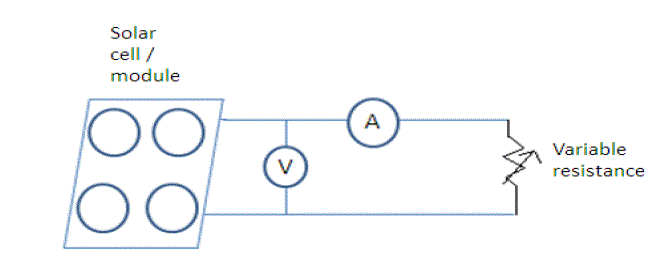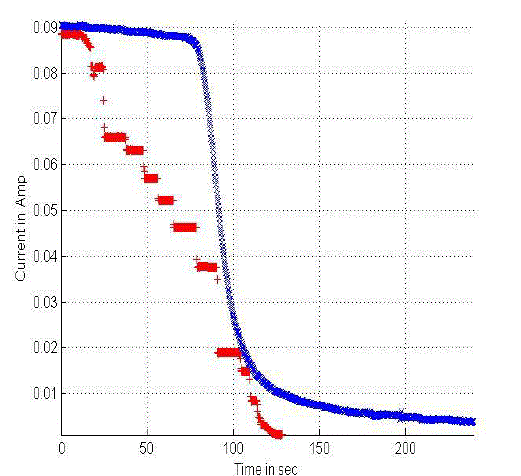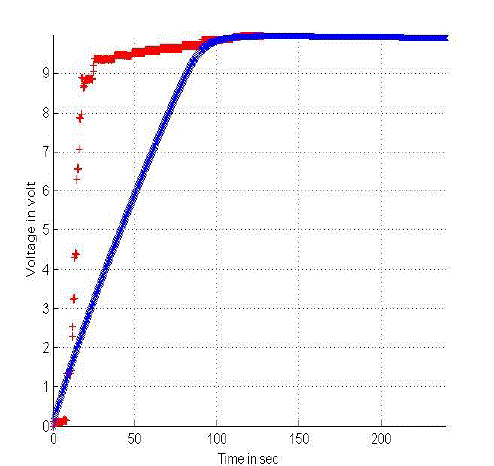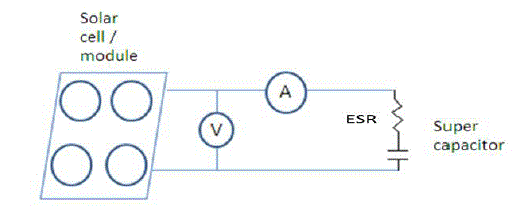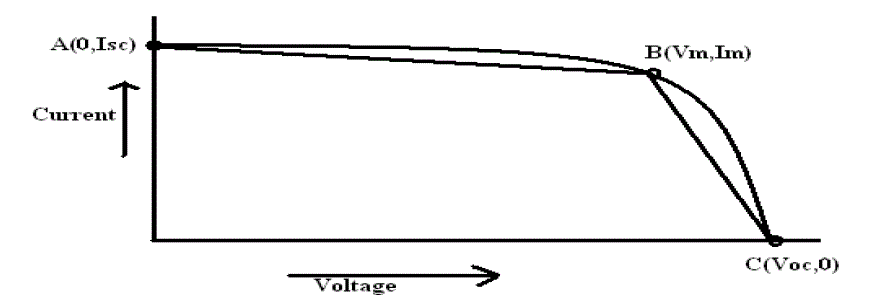Figure 1 Figure 2 Figure 3 Figure 4 Figure 5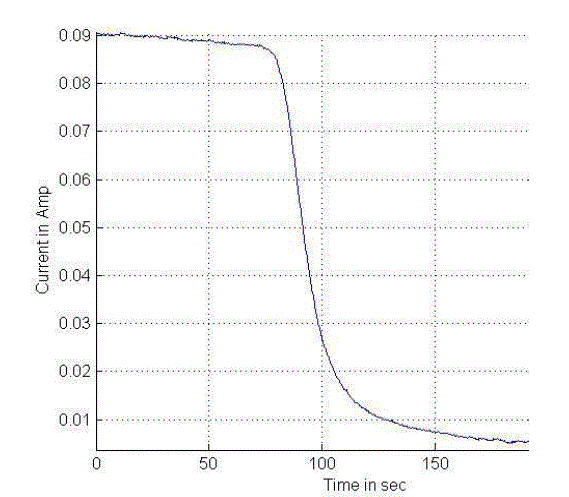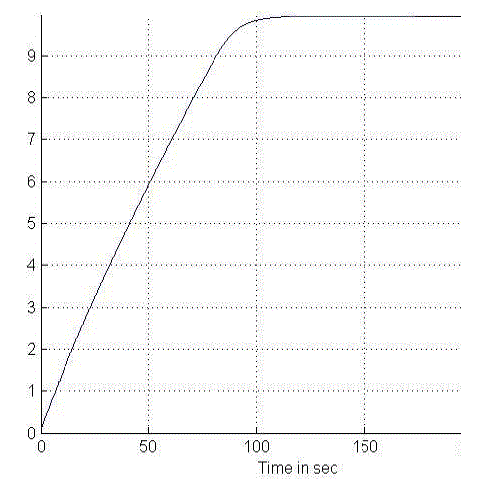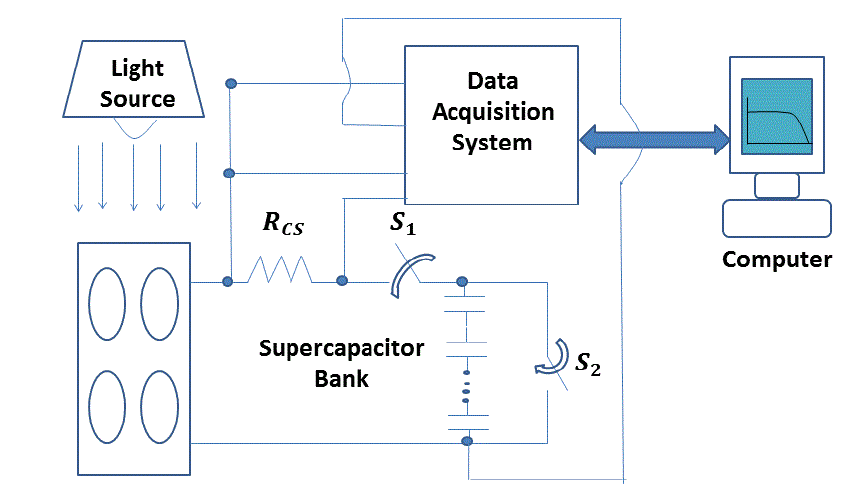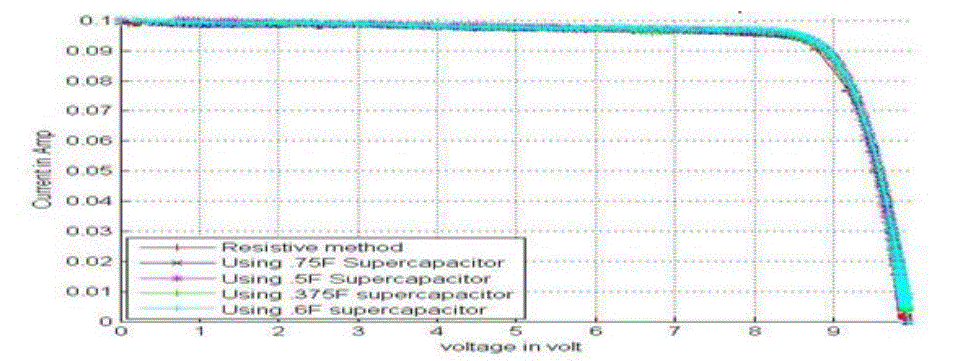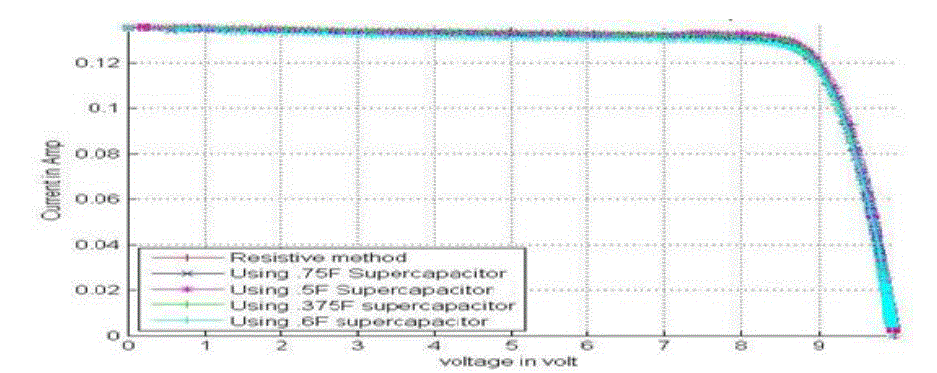Figure 5 Figure 6 Figure 7 Figure 9 Figure 10